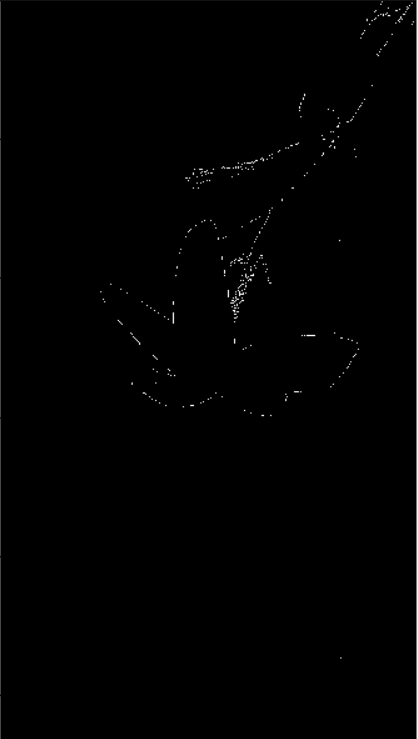# How to Perform Edge Detection in Python using OpenCV

Learning how to apply edge detection in computer vision applications using canny edge detector algorithm with OpenCV in Python.
3 min read · Updated may 2023 · Computer Vision

Turn your code into any language with our Code Converter. It's the ultimate tool for multi-language programming. Start converting now!

Disclosure: This post may contain affiliate links, meaning when you click the links and make a purchase, we receive a commission.

Edge detection is an image processing technique for finding the boundaries of objects within images. It mainly works by detecting discontinuities in brightness. One of the most popular and widely used algorithms is the Canny edge detector.

Canny edge detector is an edge detection operator that uses a multi-stage algorithm to detect a wide range of edges in images.

The main stages are:

1. Filtering out noise using the Gaussian blur algorithm.
2. Finding the strength and direction of edges using Sobel Filters.
3. Isolating the strongest edges and thinning them to one-pixel wide lines by applying non-maximum suppression.
4. Using hysteresis to isolate the best edges.

Read also: Image Transformations using OpenCV in Python.

Alright, let's implement it in Python using OpenCV, installing it:

``\$ pip install opencv-python matplotlib numpy``

Open up a new Python file and follow along:

``````import cv2
import numpy as np
import matplotlib.pyplot as plt``````

Now let's read the image when want to detect its edges:

``````# read the image

I have an example image in my current directory, make sure you do too.

Before we pass the image to the Canny edge detector, we need to convert the image to grayscale:

``````# convert it to grayscale
gray = cv2.cvtColor(image, cv2.COLOR_BGR2GRAY)``````

Let's see it:

``````# show the grayscale image
plt.imshow(gray, cmap="gray")
plt.show()``````All we need to do now is to pass this image to `cv2.Canny()` function which finds edges in the input image and marks them in the output map edges using the Canny algorithm:

``````# perform the canny edge detector to detect image edges
edges = cv2.Canny(gray, threshold1=30, threshold2=100)``````

The smallest value between `threshold1` and `threshold2` is used for edge linking. The largest value is used to find initial segments of strong edges.

Let's see the resulting image:Interesting, try to fine-tune the threshold values and see if you can make it better.

## Mastering YOLO: Build an Automatic Number Plate Recognition System

Building a real-time automatic number plate recognition system using YOLO and OpenCV library in Python

If you want to use the live camera, here is the full code for that:

``````import numpy as np
import cv2

cap = cv2.VideoCapture(0)

while True:
gray = cv2.cvtColor(frame, cv2.COLOR_BGR2GRAY)
edges = cv2.Canny(gray, 30, 100)
cv2.imshow("edges", edges)
cv2.imshow("gray", gray)
if cv2.waitKey(1) == ord("q"):
break

cap.release()
cv2.destroyAllWindows()
``````

Alright, we are done!

The purpose of detecting edges is to capture important events and changes in the properties of the world. It is one of the fundamental steps in image processing, image pattern recognition, and computer vision techniques.

Finally, I've collected some useful resources and courses for you for further learning, I highly recommend the following courses:

Learn also: How to Apply HOG Feature Extraction in Python.

Happy Learning ♥

Want to code smarter? Our Python Code Assistant is waiting to help you. Try it now!

Sharing is caring!

### Comment panel

Got a coding query or need some guidance before you comment? Check out our Python Code Assistant for expert advice and handy tips. It's like having a coding tutor right in your fingertips!